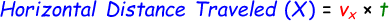# Horizontal Distance Calculator

Our online horizontal distance calculator helps you to calculate the amount of distance an object has traveled from one point to another. Here we use one of the projective motion formula to calculate which is:where,
x = Horizontal distance [meters]
vx = Velocity of the object [m/s]
t - Time taken [sec]

In the below calculator, enter the velocity of the object and time taken in the input boxes and click calculate button to find the horizontal distance.

 Velocity of the Object (vx): [m/s] Time taken (t): [sec] Horizontal Distance Traveled (x): [meters]

Latest Calculator Release

Average Acceleration Calculator

Average acceleration is the object's change in speed for a specific given time period. ...

Free Fall Calculator

When an object falls into the ground due to planet's own gravitational force is known a...

Torque Calculator

Torque is nothing but a rotational force. In other words, the amount of force applied t...

Average Force Calculator

Average force can be explained as the amount of force exerted by the body moving at giv...

Angular Displacement Calculator

Angular displacement is the angle at which an object moves on a circular path. It is de...